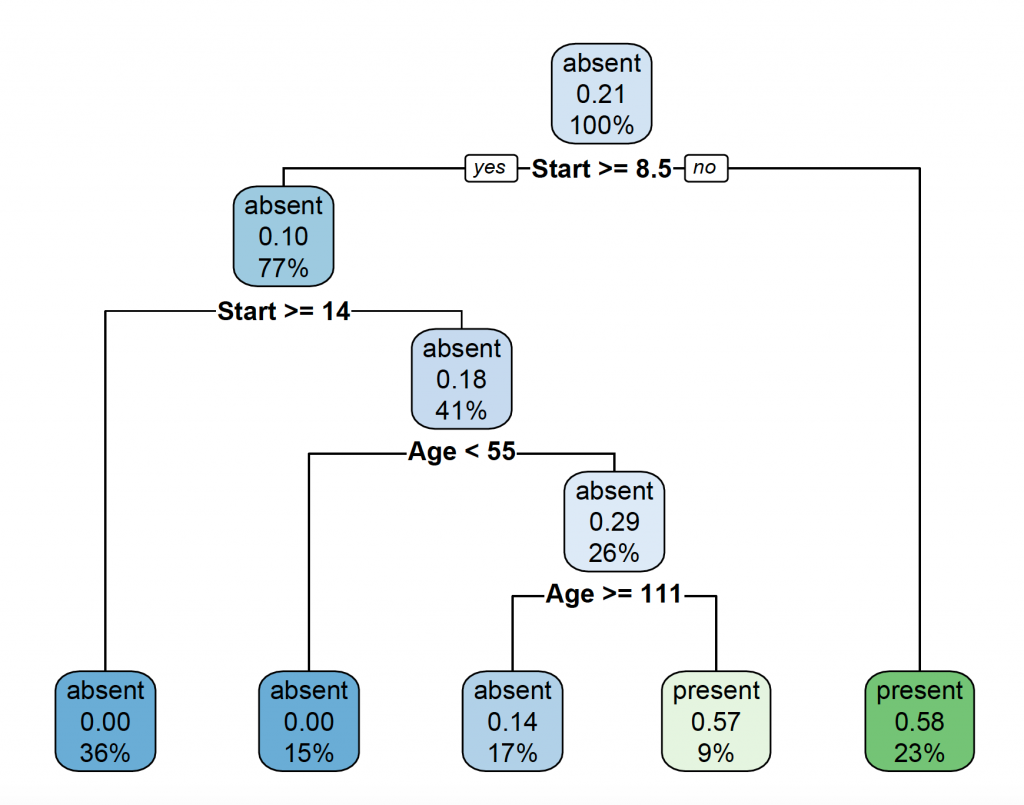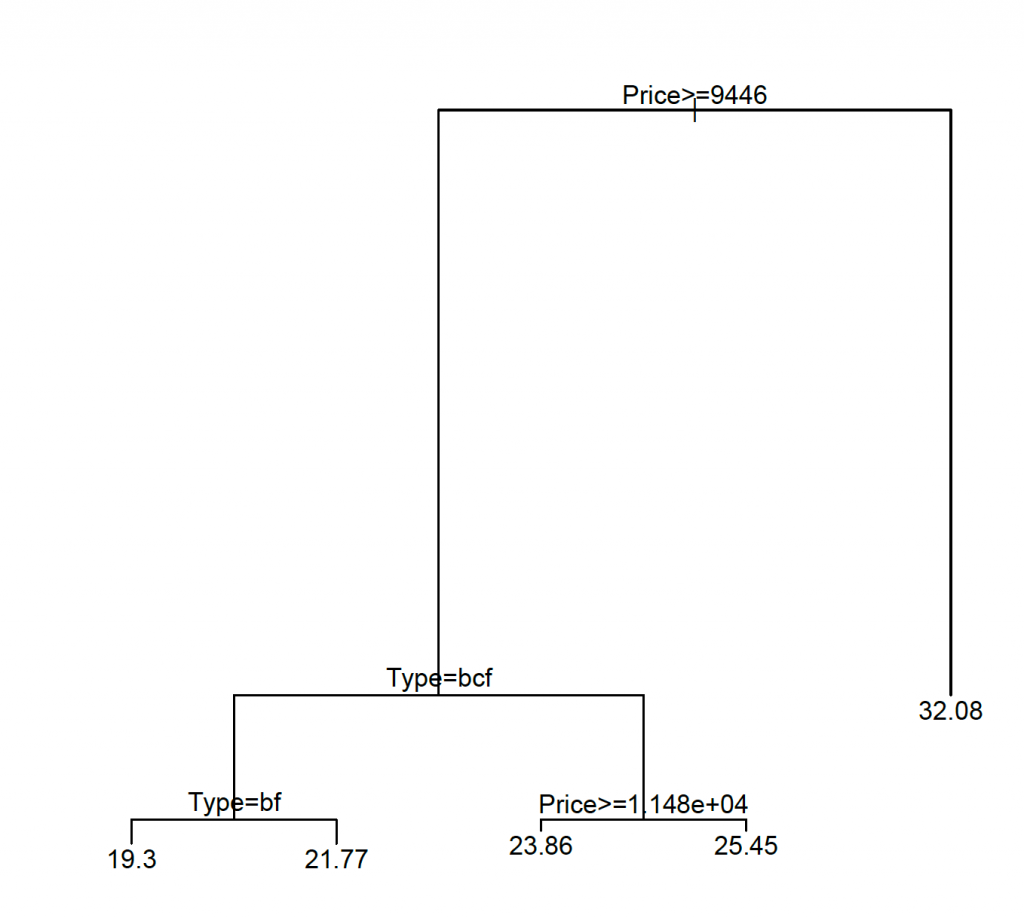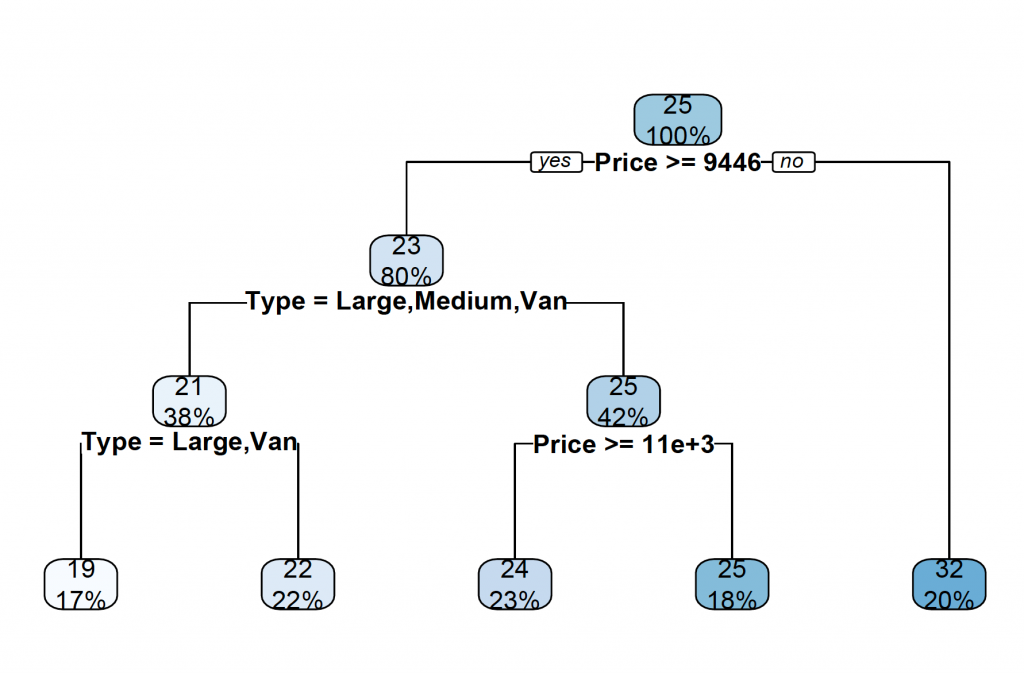# Decision Tree Plot

A decision tree plot can be useful to visualise decision rules for categorical or continuous outcome variables. A popular package to create decision trees in R is rpart 1. In addition, there is a separate package to produce plots with rpart; rpart.plot 2. Both packages should be installed.

Binary (categorical) outcome

The rpart package  1 contains a data frame with information about patients with kyphosis. To view the first 6 observations:

library(rpart)
Kyphosis Age Number Start
1 absent 71 3 5
2 absent 158 3 14
3 present 128 4 5
4 absent 2 5 1
5 absent 1 4 15
6 absent 1 2 16

The data frame contains 4 variables (str stands for structure):

str(kyphosis)
‘data.frame': 81 obs. of 4 variables:
\$ Kyphosis: Factor w/ 2 levels “absent”,”present”: 1 1 2 1 1 1 1 1 1 2 …
\$ Age : int 71 158 128 2 1 1 61 37 113 59 …
\$ Number : int 3 3 4 5 4 2 2 3 2 6 …
\$ Start : int 5 14 5 1 15 16 17 16 16 12 …

The variable ‘Kyphosis’ is categorical and is either ‘absent’ or ‘present’. The variable ‘Number’ is integer and shows the number of vertebrae involved. The variable ‘Start’ is the highest vertebra.

To create a decision tree (machine learning) plot is straight forward:

model <- rpart(Kyphosis ~ Age + Number + Start, data = kyphosis, method = ‘class’)

The variable to the left of the  tilde (~) is the outcome (response) variable and the variables to the right of the tilde are the explanatory variables. To show the model:

model
n= 81

node), split, n, loss, yval, (yprob)
* denotes terminal node

1) root 81 17 absent (0.79012346 0.20987654)
2) Start>=8.5 62 6 absent (0.90322581 0.09677419)
4) Start>=14.5 29 0 absent (1.00000000 0.00000000) *
5) Start< 14.5 33 6 absent (0.81818182 0.18181818)
10) Age< 55 12 0 absent (1.00000000 0.00000000) *
11) Age>=55 21 6 absent (0.71428571 0.28571429)
22) Age>=111 14 2 absent (0.85714286 0.14285714) *
23) Age< 111 7 3 present (0.42857143 0.57142857) *
3) Start< 8.5 19 8 present (0.42105263 0.57894737) *

plot(model)
text(model)Although it is possible to reduce the text size (with cex = 0.8), the plot doesn’t look very good. However, there is an additional package rpart.plot2 for enhanced graphics.

library(rpart.plot)
rpart.plot(model)Continuous outcome variable (regression)

The rpart package also contains a data frame cu.summary with information about different cars. To show the data frame’s first six observations as well as its structure:

library(rpart)
Price Country Reliability Mileage Type
Acura Integra 4 11950 Japan Much better NA Small
Dodge Colt 4 6851 Japan <NA> NA Small
Dodge Omni 4 6995 USA Much worse NA Small
Eagle Summit 4 8895 USA better 33 Small
Ford Escort 4 7402 USA worse 33 Small
Ford Festiva 4 6319 Korea better 37 Small
str(cu.summary)
‘data.frame': 117 obs. of 5 variables:
\$ Price : num 11950 6851 6995 8895 7402 …
\$ Country : Factor w/ 10 levels “Brazil”,”England”,..: 5 5 10 10 10 7 5 6 6 7 …
\$ Reliability: Ord.factor w/ 5 levels “Much worse”<“worse”<..: 5 NA 1 4 2 4 NA 5 5 2 …
\$ Mileage : num NA NA NA 33 33 37 NA NA 32 NA …
\$ Type : Factor w/ 6 levels “Compact”,”Large”,..: 4 4 4 4 4 4 4 4 4 4 …

To predict the car’s mileage from Price, Country, Reliability and Type (using analysis of variance: anova):

model2 <- rpart(Mileage ~ Price + Country + Reliability + Type, method=”anova”, data=cu.summary)
plot(model2)
text(model2)Or for a nicer plot:

library(rpart.plot)
rpart.plot(model2)1.
Therneau T, Atkinson B, Ripley B. rpart: Recursive Partitioning and Regression Trees [Internet]. 2017. Available from: https://cran.r-project.org/web/packages/rpart/index.html
1.
Milborrow S. rpart.plot: Plot “rpart” Models: An Enhanced Version of “plot.rpart” [Internet]. 2017. Available from: https://cran.r-project.org/web/packages/rpart.plot/index.html